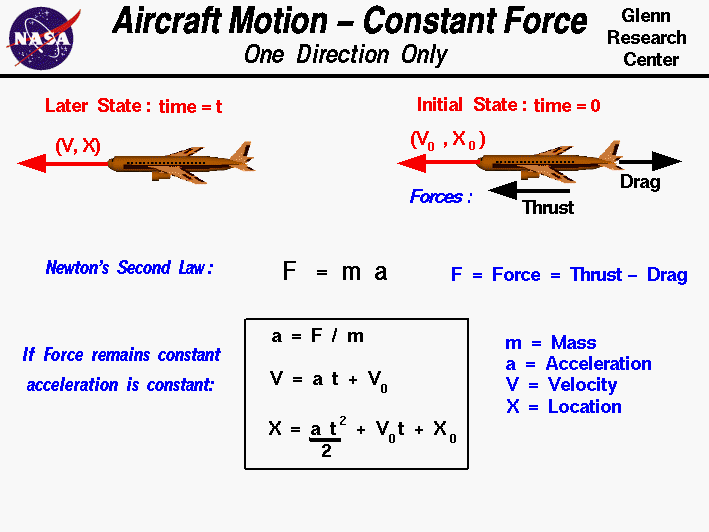+ Text Only Site
+ Non-Flash Version
+ Contact GlennAs discussed on the airplane cruise slide, an airplane can maintain a constant speed and level flight in which the weight is balanced by the lift, and the drag is balanced by the thrust. However, if the forces become unbalanced, the aircraft moves in the direction of the greater force. We can compute the acceleration of the aircraft from Newton's Second Law of Motion. If the mass m of the aircraft remains a constant we can use the familiar form of the equation to solve for the acceleration a F = m * a a = F / m where the net force F is the difference between the opposing forces; lift minus weight or thrust minus drag. The mass m of the aircraft is determined by the weight of the aircraft. If the force and the mass remain constant, the basic equations of motion can be solved. For a constant force and constant mass, the acceleration remains constant. The velocity V at any time t is the acceleration times the time, plus the initial velocity Vo: V = a * t + Vo Similarly, the location X at any time t is given by 1/2 the acceleration times the time squared, plus the initial location Xo, plus the initial velocity times the time. X = .5 * a * t^2 + Vo * t + Xo Note that these equations can be used only if the mass and the force are constant. The mass of an aircraft remains fairly constant during cruise since the only loss is for the fuel which is consumed. Fuel mass is normally a small percentage of the mass of an aircraft. However for aircraft, the lift and drag forces are themselves functions of the square of the velocity. So we can assume a constant force for only a very small amount of time. To solve the actual equations of motion for an aircraft, we must use calculus and integrate the equations of motion. The integration can be performed analytically or numerically. Also note that on this slide we solve the equations of motion in only the x-direction. The forces, accelerations, velocities and displacements are all vector quantities which are defined in three spatial directions and time. The x-direction is only one component of the more complex three-dimensional motion. An interactive Java applet that demonstrates the information found on this slide is also available at this web site. The applet presents problems that you must solve by using the equations of motion. Activities:Guided ToursBasic Aircraft Motion:RangeGames:Gradual Climb:Navigation ..Beginner's Guide Home Page+ Inspector General Hotline + Equal Employment Opportunity Data Posted Pursuant to the No Fear Act + Budgets, Strategic Plans and Accountability Reports + Freedom of Information Act + The President's Management Agenda + NASA Privacy Statement, Disclaimer, and Accessibility CertificationEditor: Nancy Hall NASA Official: Nancy Hall Last Updated: May 13 2021 + Contact Glenn